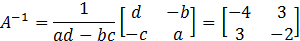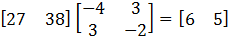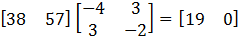### Sample Problem

Use the inverse of the matrixto decode the cryptogram:

51 72 27 38 27 36 38 57 59 81 42 61

Divide the message into groups of two (the matrix A is a 2 x 2) to form the coded row matrices and multiply each coded row matrix by A-1 on the right.

#### Solution

Find A-1Divide the message into groups of two (the matrix A is a 2 x 2) to form the coded row matrices and multiply each coded row matrix by A-1 on the right.Thus, the message is: